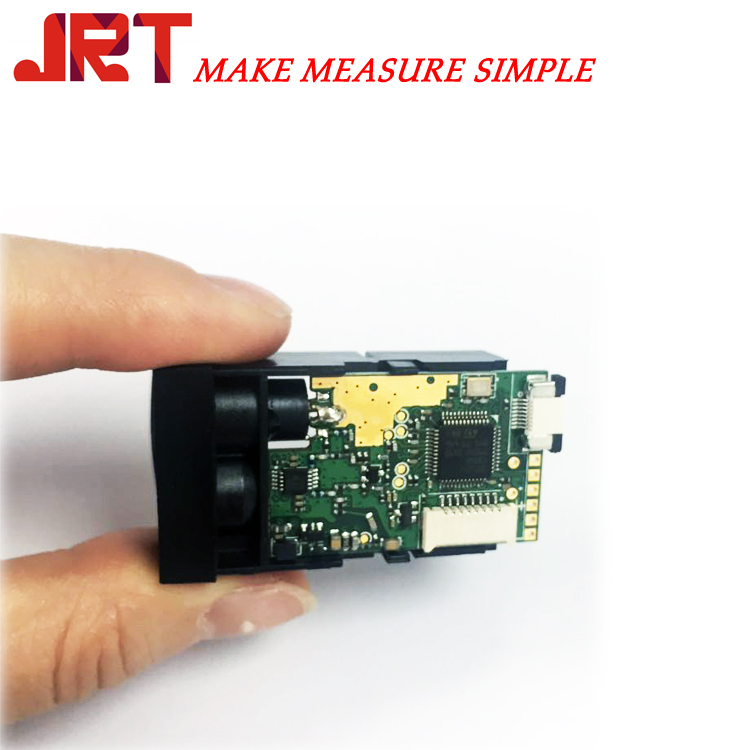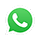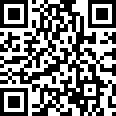Kommunicera med leverantören? LeverantörMs. Amanda Cao
Vad kan jag göra för dig?

Industriinformation

Home > Industriinformation > Laser rangefinder

# Laser rangefinder

2019-01-21

[ Pacific Security Network News ]
As a high-precision measuring instrument, laser range finder has been widely used in people's lives. However, when choosing a laser range finder, the price of the laser range finder is quite different. Why is this? Let's take a look at the laser rangefinder price information.
There are many factors that affect the price of laser range finder. If you do not consider the brand value, you should first consider the type of laser range finder.
Laser range finder is divided into two categories, one is handheld laser range finder, such range finder measuring distance is relatively short, generally 40-300 meters, high measurement accuracy. The other type is the laser range finder telescope. This kind of laser range finder measures a long distance, generally 500-2000 meters, and the longest measuring distance can reach 20 kilometers.
First, the price of handheld laser range finder
Handheld laser range finder is currently not on the market, the price of handheld laser range finder generally ranges from 600-4000 yuan.
The main factors affecting the price of handheld laser rangefinders:
1. Measuring distance: The measuring distance of the handheld laser range finder is generally 40-300 meters. The farther the measuring distance is, the higher the price is. The price of the handheld laser range finder below 50 meters is generally less than 1000 yuan.
2. Function: There are many functions of the handheld laser range finder. It is necessary to know in detail when purchasing. The more functions, the more expensive the price will be.
Second, the price of telescope laser range finder
Telescope laser range finder, the world's top four brands are Tuyad, PhD, Olfa and Nikon. There are also some domestic brands. The telescope-type laser range finder generally measures from 500 to 2000 meters, and there is also a 20KM ultra-long-range telescope laser range finder that sells for more than 100,000. Here we mainly introduce the commonly used telescope laser range finder of 50-2000 meters.
In general, the price of a telescope laser range finder can be roughly classified in this way.
1. Only the distance measurement model
Can not measure height, angle measurement, only the range function. 500-800 meters, the price is about 800-1500 yuan, 800-1200 meters price is about 1500-2500 yuan, the price of 1200-200 meters is about 2500-4000 yuan.
2. Multi-function telescope laser range finder
According to different functions, there will be differences in price. The main functions are whether there are height measurement, angle measurement, horizontal distance measurement, height difference (absolute height), continuous distance measurement, continuous angle measurement (no need to trigger infrared emission light) )Wait.
Main functional factors affecting the price of telescope laser range finder
1. Measuring distance: This is the main indicator of the laser range finder telescope. It refers to the telescope's farthest measurement. The distance measured by the telescope is generally 400-2000 meters. This distance refers to the linear distance from the measurement position to the measurement target. There is also an important indicator behind it, which is the horizontal distance (the two concepts must not be confused). The measurement distance of a laser rangefinder telescope is generally a theoretical measurement distance and will be affected by the weather. Therefore, when choosing a range finder, you should in principle choose a laser rangefinder telescope that is farther than the farthest measurement distance you actually require. Measuring distance is the most important factor affecting the price of telescope laser range finder.
2. Whether it can measure height: Whether it can measure the level, many rangefinders have this function, so this range finder can also be called altimeter. Another concept of altimetry is to measure the absolute height of the target (measured height difference). These two concepts must not be confused. At present, the brands that can be measured on the market are mainly Tuyad and Orfa, and the Nikon 1000AS can also measure the height, but it cannot measure the angle. All models of the doctor can not measure high. The model that can measure high, the price will be more expensive than the model without this function.
3. Can the angle measurement: Whether it can measure the angle, many rangefinders also have this function, so this range finder can also be called the goniometer. Many of Turda and Orfa's models have this feature, and all Nikon models cannot measure angles. Some models of the doctor can measure the angle. The ability to measure angles is another major factor affecting the price of telescope laser rangefinders.
4. Can measure the absolute height of the target (measured height difference): Unlike the height measurement concept mentioned above, it refers to the height of the measurement target itself, which is the function of the high-end range finder. At present, only the SP1500H of Tuya has this advanced function. However, in the new products released by Alpha in Germany in May 2012, there is a rangefinder with such functions. Both Dr. and Nikon do not have such a model.
5. Can measure horizontal distance: The measured distance mentioned above refers to the linear distance from the measurement position to the measurement target, and the horizontal distance refers to the horizontal distance from the measurement position to the measurement target. These two distances are different. This is a feature of the high-end rangefinder.
6. Can continuous ranging: Continuous ranging is very useful in many situations, so this function is also very important.
7. Can continuous angle measurement: The function of continuous angle measurement will have this function in some high-end laser rangefinder telescopes. Continuous angle measurement means continuous angle measurement without triggering an infrared emission lamp. Currently only the Tuyad SP1500H has this feature.
The above is the price information of the laser range finder summarized by Xiaobian and the factors affecting the price of the laser range finder. More information about the laser range finder.

Skicka förfråganMs. Amanda Cao

Tel:86-28-68731517/62634110/68731157

Fax:86-28-68730057

Mobiltelefon:+8615982044032E-post:sales@jrt-measure.com

Adress: 7-1-1510, W6, New Century Global Center, Gaoxin District, Chengdu, Sichuan

Mobile Site